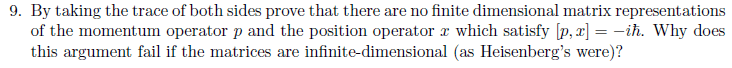# Trace of Position and Momentum Operator

• unscientific

## Homework Statement

Hi guys, I've not started a course on QM yet, but we are currently learning the maths used in QM.

Show, by taking the trace of both sides show that finite dimensional matrix representations
of the momentum operator p and the position operator x which satisfy [p, x] = −ih are not possible.

Why does this argument fail if the matrices are infinite-dimensional (as Heisenberg’s were)?## The Attempt at a Solution

Are the position and momentum operators supposed to be matrices? I've looked up on wikipedia, the position operator is more of like ih(d/dp) while the momentum operator is ih(d/dx).

Last edited: